# OpenCV 直方图均衡化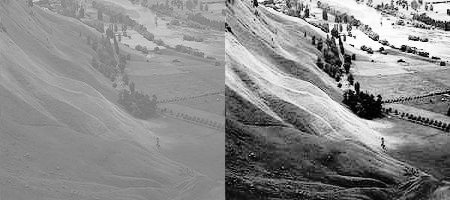## 直方图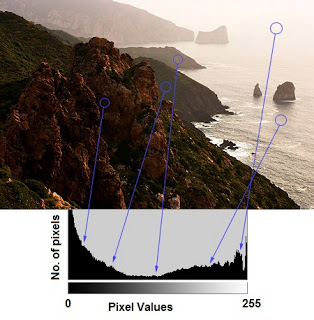### 直方图相关术语

#### BINS

BINS：上面的直方图显示了每个像素值的像素数，从0到255。您需要256个值来显示以上的直方图。但是，考虑一下，如果您不需要单独查找所有像素值的像素数量，而是在一个像素值区间内的像素数量，该怎么办？例如，你需要找到介于0到15之间的像素数，然后是16到31……240到255。您只需要16个值来表示这个直方图。OpenCV Tutorials on histograms中展示了这个例子。

#### DIMS

DIMS：它是我们收集数据的参数的个数。在这种情况下，我们收集的数据只有一件事，强度值。所以这里是1。

#### RANGE

RANGE：它是你想测量的强度值的范围。通常，它是 [ 0，256 ]，也就是所有的强度值。

### OpenCV中直方图的计算

OpenCV提供了cv.calcHist()函数来获取直方图。让我们熟悉一下这个函数及其参数：

cv.calcHist(images, channels, mask, histSize, ranges[, hist[, accumulate]])
• images：它是uint8类型或float32的源图像。它应该用方括号括起来，也就是”[img]”。

• channels：它也用方括号括起来。它是我们计算直方图的信道的索引。例如，如果输入是灰度图像，它的值是0。对于颜色图像，您可以通过0、1或2来分别计算蓝色、绿色或红色通道的直方图。

• histSize：这代表了我们的BINS数。需要用方括号来表示。在整个范围内，我们通过了256。

• ranges：强度值范围，通常是 [ 0，256 ]

img = cv.imread('home.jpg', 0)
hist = cv.calcHist([img], , None, , [0,256])

hist是一个256x1阵列，每个值对应于该图像中的像素值及其对应的像素值。

### Numpy中直方图的计算

Numpy中提供了np.histogram()方法,用于对一维数组进行直方图统计，其参数列表如下：

Histogram(a,bins=10,range=None,normed=False,weights=None)
• a：是保存待统计的数组

• bins：指定统计的区间个数，即对统计范围的等分数

• range：是一个长度为2的元组，表示统计范围的最大值和最小值，默认值为None，表示范围由数据的范围决定，即(a.min(), a.max))。

• normed：当normed参数为False时，函数返回数组a中的数据在每个区间的个数，否则对个数进行正规化处理，使它等于每个区间的概率密度。

• weights：weights参数和 bincount()的类似

• hist : hist和之前计算的一样，每个区间的统计结果。

• bins : 数组，存储每个统计区间的起点。range为[0,256]时，bins有257个元素，因为Numpy计算bins是以0-0.99,1-1.99等，所以最后一个是255-255.99。为了表示这一点，他们还在bins的末端添加了256。但我们不需要256。到255就足够了。

hist, bins = np.histogram(img.ravel(), 255, [0,256])

Numpy还有另一个函数，np.bincount()，比np.histograme()要快得多(大约10X)。对于一维直方图，你可以试一下。不要忘记在np.bincount中设置minlength=256。例如，hist=np.bincount(img.ravel()，minlength=256)

OpenCV函数比np.histogram()快（大约40X）。所以考虑效率的时候坚持用OpenCV函数。

## 绘制直方图

### 1. 使用Matplotlib

Matplotlib有一个绘制直方图的函数：

matplotlib.pyplot.hist()

import numpy as np
import cv2 as cv
from matplotlib import pyplot as plt

plt.hist(img.ravel(), 256, [0,256])
plt.show()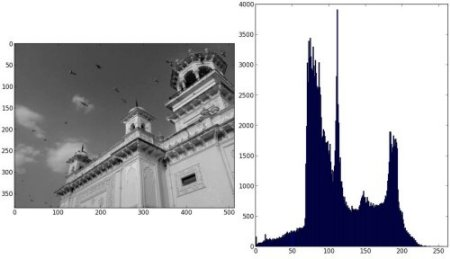import numpy as np
import cv2 as cv
from matplotlib import pyplot as plt

color = ('b', 'g', 'r')
for i, col in enumerate(color):
histr = cv.calcHist([img], [i], None, , [0,256])
plt.plot(histr, color=col)
plt.xlim([0,256])
plt.show()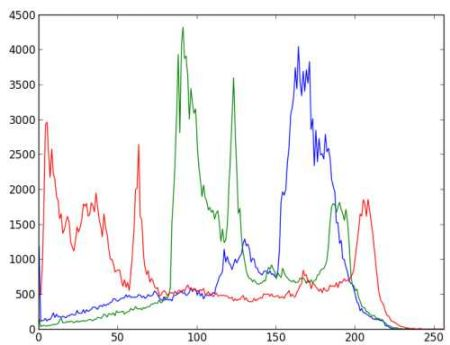### 2. 使用OpenCV

#### 应用遮罩

img = cv.imread('home.jpg', 0)

hist_full = cv.calcHist([img], , None, , [0,256])

plt.subplot(221), plt.imshow(img, 'gray')
plt.xlim([0,256])

plt.show()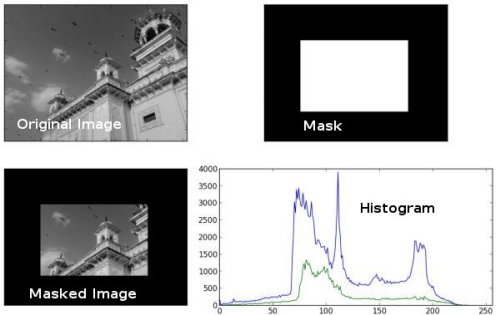## 直方图均衡化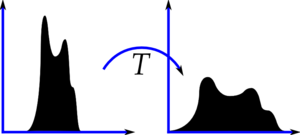### 直方图均衡化算法

from 《计算机视觉-算法与应用》 Richard Szeliski

c(I) = \frac{1}{N}\sum_{i=0}^{I}h(i) = c(I-1)+\frac{1}{N}h(I)### Numpy中的直方图均衡化

#### Numpy相关函数

##### 计算累计和cumsun()
numpy.cumsum(a, axis=None, dtype=None, out=None)

>>>import numpy as np
>>> b=[1,2,3,4,5,6,7]
>>> np.cumsum(a)
array([  1,   3,   6,  10,  15,  21,  28,  36,  45,  55,  75, 105])

#### 直方图均衡化

import numpy as np
import cv2 as cv
from matplotlib import pyplot as plt

hist, bins = np.histogram(img.flatten(), 256, [0,256])

cdf = hist.cumsum()
cdf_normalized = cdf*float(hist.max())/cdf.max()

plt.plot(cdf_normalized, color = 'b')
plt.hist(img.flatten(),256,[0,256], color = 'r')
plt.xlim([0,256])
plt.legend(('cdf','histogram'), loc = 'upper left')
plt.show()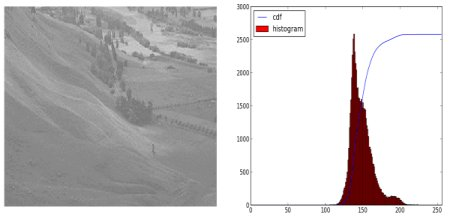cdf = (cdf-cdf) *255/ (cdf[-1]-1)
cdf = cdf.astype(np.uint8)

img2 = cdf[img]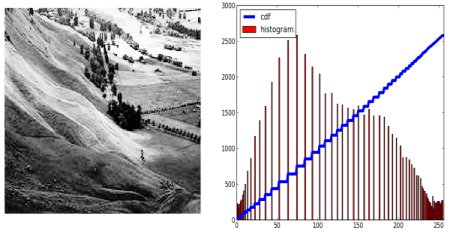#### 示例1：单通道的灰阶图的直方图均衡化

import cv2 as cv
import numpy as np
from matplotlib import pyplot as plt

cv.imshow("before", img)

# calculate hist
hist, bins = np.histogram(img, 256)
# calculate cdf
cdf = hist.cumsum()
# plot hist
plt.plot(hist,'r')

# remap cdf to [0,255]
cdf = (cdf-cdf)*255/(cdf[-1]-1)
cdf = cdf.astype(np.uint8)# Transform from float64 back to unit8

# generate img after Histogram Equalization
img2 = np.zeros((384, 495, 1), dtype =np.uint8)
img2 = cdf[img]

hist2, bins2 = np.histogram(img2, 256)
cdf2 = hist2.cumsum()
plt.plot(hist2, 'g')

cv.imshow("after", img2)
plt.show()
cv.waitKey(0)#### 示例2：彩图的直方图均衡化

import cv2 as cv
import numpy as np
from matplotlib import pyplot as plt

cv.imshow("before", img)

# split g,b,r
g = img[:,:,0]
b = img[:,:,1]
r = img[:,:,2]

# calculate hist
hist_r, bins_r = np.histogram(r, 256)
hist_g, bins_g = np.histogram(g, 256)
hist_b, bins_b = np.histogram(b, 256)

# calculate cdf
cdf_r = hist_r.cumsum()
cdf_g = hist_g.cumsum()
cdf_b = hist_b.cumsum()

# remap cdf to [0,255]
cdf_r = (cdf_r-cdf_r)*255/(cdf_r[-1]-1)
cdf_r = cdf_r.astype(np.uint8)# Transform from float64 back to unit8
cdf_g = (cdf_g-cdf_g)*255/(cdf_g[-1]-1)
cdf_g = cdf_g.astype(np.uint8)# Transform from float64 back to unit8
cdf_b = (cdf_b-cdf_b)*255/(cdf_b[-1]-1)
cdf_b = cdf_b.astype(np.uint8)# Transform from float64 back to unit8

# get pixel by cdf table
r2 = cdf_r[r]
g2 = cdf_g[g]
b2 = cdf_b[b]

# merge g,b,r channel
img2 = img.copy()
img2[:,:,0] = g2
img2[:,:,1] = b2
img2[:,:,2] = r2

# show img after histogram equalization
cv.imshow("img2", img2)

cv.waitKey(0)#### 示例3：带遮罩的直方图均衡化

import cv2 as cv
import numpy as np
from matplotlib import pyplot as plt

cv.imshow("src", img)

# calculate hist
hist, bins = np.histogram(masked_img.compressed(), 256) # img have to be compressed() to let mask work
# calculate cdf
cdf = hist.cumsum()

print(cdf)
# plot hist
plt.plot(hist,'r')

# remap cdf to [0,255]
cdf = (cdf-cdf)*255/(cdf[-1]-1)
cdf = cdf.astype(np.uint8)# Transform from float64 back to unit8

# generate img after Histogram Equalization
img2 = np.zeros((384, 495, 1), dtype =np.uint8)
img2 = cdf[img]

hist2, bins2 = np.histogram(img2, 256)
cdf2 = hist2.cumsum()
plt.plot(hist2, 'g')

cv.imshow("dst", img2)
plt.show()
cv.waitKey(0)How to create the histogram of an array with masked values, in Numpy?

### OpenCV中的直方图均衡化

OpenCV有一个函数可以这样做，cv.equalizeHist()，它封装好了计算cdf和cdf重映射以及根据cdf表生成直方图均衡图像的过程。它的输入只是灰度图像，输出是我们的直方图均衡图像。

img = cv.imread('wiki,jpg', 0)
equ = cv.equalizeHist(img)
res = np.hstack((img, equ)) # 并排叠加图片
cv.imwrite('res.png', res)#### 示例1：单通道的灰阶图的直方图均衡化

import cv2 as cv
import numpy as np
from matplotlib import pyplot as plt

cv.imshow("before", im)

# Histogram Equalization
im2 = cv.equalizeHist(im)
print(im2)

cv.imshow("after", im2)
plt.show()
cv.waitKey(0)

#### 示例2：彩图的直方图均衡

import cv2 as cv
import numpy as np
from matplotlib import pyplot as plt

cv.imshow("before", im)

# split g,b,r
g = im[:,:,0]
b = im[:,:,1]
r = im[:,:,2]

# Histogram Equalization
r2 = cv.equalizeHist(r)
g2 = cv.equalizeHist(g)
b2 = cv.equalizeHist(b)

im2 = im.copy()
im2[:,:,0] = g2
im2[:,:,1] = b2
im2[:,:,2] = r2

print(im2)

cv.imshow("after", im2)
plt.show()
cv.waitKey(0)

#### 示例3：带遮罩的直方图均衡化

import cv2 as cv
import numpy as np
from matplotlib import pyplot as plt

cv.imshow("before", im)

# Histogram Equalization
cdf = (cdf-cdf)*255/(cdf[-1]-1)
cdf = cdf.astype(np.uint8)# Transform from float64 back to unit8

# generate img after Histogram Equalization
im2 = np.zeros((384, 495, 1), dtype =np.uint8)
im2 = cdf[im]

# im2 = cv.equalizeHist(im)
print(im2)

cv.imshow("after", im2)
plt.show()
cv.waitKey(0)### CLAHE（对比有限的自适应直方图均衡/Contrast Limited Adaptive Histogram Equalization）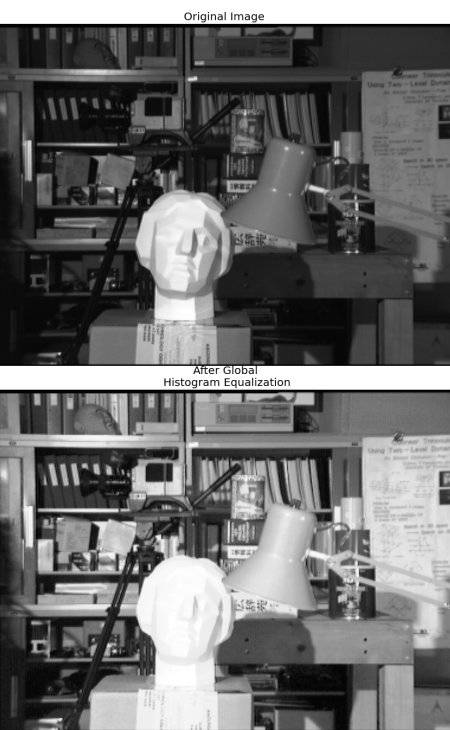cv.createCLAHE([, clipLimit[, tileGridSize]])
import numpy as np
import cv2 as cv

# create a CLAHE object (Arguments are optional).
clahe = cv.createCLAHE(clipLimit=2.0, tileGridSize=(8,8))
cl1 = clahe.apply(img)

cv.imread('clahe_2.jpg', cl1)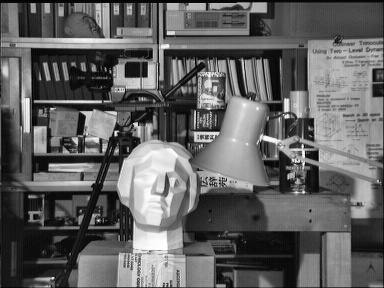02-0111-039051
06-253423
01-151452
09-29471
12-18
07-031万+
04-091670
05-283805
07-19205
09-231779
01-051960
01-28832
05-08404
04-191404
09-156246
07-261468
05-201万+
03-301006